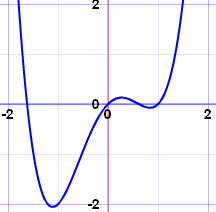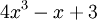# Polynomials

A polynomial looks like this:example of a polynomial this one has 3 terms

Polynomial comes from poly- (meaning "many") and -nomial (in this case meaning "term") ... so it says "many terms"

A polynomial can have:

 constants (like 3, −20, or ½) variables (like x and y) exponents (like the 2 in y2), but only 0, 1, 2, 3, ... etc are allowed

that can be combined using addition, subtraction, multiplication and division ...

... except ...

 ... not division by a variable (so something like 2/x is right out)

So:

A polynomial can have constants, variables and exponents,
but never division by a variable.

Also they can have one or more terms, but not an infinite number of terms.

## Polynomial or Not?These are polynomials:

• 3x
• x − 2
• −6y2 − ( 7 9 )x
• 3xyz + 3xy2z − 0.1xz − 200y + 0.5
• 512v5 + 99w5
• 5

(Yes, "5" is a polynomial, one term is allowed, and it can be just a constant!)

These are not polynomials

• 3xy-2 is not, because the exponent is "-2" (exponents can only be 0,1,2,...)
• 2/(x+2) is not, because dividing by a variable is not allowed
• 1/x is not either
• √x is not, because the exponent is "½" (see fractional exponents)

But these are allowed:

• x/2 is allowed, because you can divide by a constant
• also 3x/8 for the same reason
• √2 is allowed, because it is a constant (= 1.4142...etc)

## Monomial, Binomial, Trinomial

There are special names for polynomials with 1, 2 or 3 terms:How do you remember the names? Think cycles!There is also quadrinomial (4 terms) and quintinomial (5 terms),
but those names are not often used.

## Variables

Polynomials can have no variable at all

Example: 21 is a polynomial. It has just one term, which is a constant.

Or one variable

Example: x4 − 2x2 + x   has three terms, but only one variable (x)

Or two or more variables

Example: xy4 − 5x2z   has two terms, and three variables (x, y and z)

## What is Special About Polynomials?

Because of the strict definition, polynomials are easy to work with.

For example we know that:

So you can do lots of additions and multiplications, and still have a polynomial as the result.

Also, polynomials of one variable are easy to graph, as they have smooth and continuous lines.

### Example: x4−2x2+xSee how nice and smooth the curve is?

You can also divide polynomials (but the result may not be a polynomial).

## Degree

The degree of a polynomial with only one variable is the largest exponent of that variable.

### Example:The Degree is 3 (the largest exponent of x)

For more complicated cases, read Degree (of an Expression).

## Standard Form

The Standard Form for writing a polynomial is to put the terms with the highest degree first.

### Example: Put this in Standard Form: 3x2 − 7 + 4x3 + x6

The highest degree is 6, so that goes first, then 3, 2 and then the constant last:

x6 + 4x3 + 3x2 − 7

You don't have to use Standard Form, but it helps.